# Pre-trained Word Embeddings or Embedding Layer: A Dilemma

Source: Deep Learning on Medium

## A comparison between the effects of pre-trained word embeddings and embedding layers in the performance of semantic NLP tasks

When word embeddings became available almost a decade ago, they changed Natural Language Processing (NLP) forever. As Ronan Colobert et al. in their famous 2008 JMLR paper put it, they caused NLP to be redeveloped “almost from scratch”. A few years later, in 2013, with the release of Mikolov et al. (2013)’s word2vec library, they quickly became the dominant approach for vectorizing textual data. The NLP models that were already well studied based on traditional approaches such as LSI and TF-IDF were being put to the test against word embeddings and in most cases, word embeddings came out on top. Many embedding approaches have been developed since. Moreover, major Deep Learning (DL) packages incorporate an embedding layer that learns task-specific embeddings.

While I found several studies that compare the performance of different types of pre-trained word embeddings, I could not find any comprehensive research that compares the performance of pre-trained word embeddings to the performance of an embedding layer. This, however, is one of the first questions that I ask myself when I start implementing a new DL model. To answer this question, I carried out several experiments in order to compare the impacts of pre-trained word embeddings and an embedding layer on the performance of a DL model on two semantic tasks i.e. Sentiment Analysis and Question Classification. But first, let’s review the underlying idea behind word embeddings and have a brief look at their history.

### Judge a Word by the Company it Keeps

Why word embeddings work better than traditional word vectors is outside the scope of this article. However, they are based on a rich theory from Linguistics called Distributional Hypothesis, devised in 1950s. The theory defines the semantics of a word by looking at its context.

“You shall know a word by the company it keeps.” — John R. Firth (a dominant figure in 20th century Linguistics)

Lin (1998) exemplifies this concept with the word Tezgüino that is a rare proper noun, unfamiliar to many. It, however, becomes easy to infer as soon as we see it in the context: “A bottle of Tezgüino is on the table. Tezgüino makes you drunk’’. In other words, even if you don’t know the meaning of tezgüino, its context clarifies its meaning.

### A Brief History of Word Embeddings

Traditionally, many statistical and rule-based models were proposed, attempting to model the distributional semantics. Predominantly, a concurrence matrix was created for all of the words that appeared in a corpus and then this matrix was projected into a much smaller space using methods such as LSA. Detailed discussion of the traditional models are outside the scope of this article, however, it can provide valuable insights into the semantics of words and phrases and the underlying concepts of unsupervised word vectors. For related work, see Mitchell and Lapata (2008), Katrin Erk (2010), and Katz and Giesbrecht (2006).

It was not until 2003 when the idea of learning semi-supervised word embeddings was investigated by Yoshua Bengio et al. (2003). In their work, the authors present a neural network that learns a probability function for word sequences (the main goal of a language model) and simultaneously learns a distributional representation for each word. They introduce the weights of the input to the hidden layer of the neural network as word embeddings. Their model is, however, computationally expensive and far from practical, mainly due to the Softmax output layer. The objective of the model is to learn a function that predicts a good conditional probability distribution given an observed word sequence:

The architecture of the network can be seen below: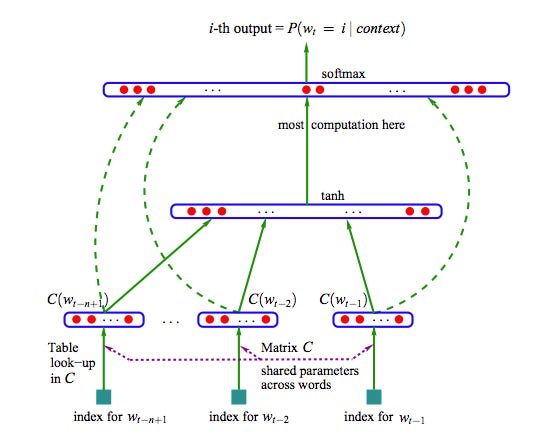Figure from Bengio et al. 2003. The proposed architecture for learning word representations.

where C(i) is the representation for the i^th word of the vocabulary. The output layer — which causes the model to be impractical, calculates the conditional probability distribution over the entire vocabulary for each word:

where N is the network before the Softmax layer. This computational complexity issue of the model was addressed by Collobert et al. (2008) who present a convolutional neural network that uses a different objective function. Unlike Bengio et al. (2003), their main concern is not language modeling but to learn good word embeddings. Hence, they have more freedom to reach this goal. They benefit from the context before and after the word for which the representation is being learned. Although this model produces the (first) unsupervised word embeddings that incorporate semantic and syntactic information, it is still computationally expensive. Due to the high computational costs of previous models, it was not until 2013 when Mikolov et al. (2013) present their simple, yet efficient model that popularised word embeddings and it begin to permeate through NLP.

They propose two architectures to compute word embeddings: Continuous Bag-of-Words (CBOW) and Skip-gram (SG) models. The CBOW model where context (w_{i-2}, w_{i-1}, w_{i+1}, w_{i+2}) is used to predict the target word (w_i), is illustrated in the following Figure:

The SG model on the other hand, tries to predict the context from a given word. As seen above, the CBOW model of Mikolov et al. (2013) has a much simpler architecture compared to the previous work which led to the low computational costs of this model. The implementation of this model (word2vec) facilitated widespread experiments with word embeddings across different areas in NLP. The experiments that showed the use of word embeddings, led to improvement in the majority of NLP tasks (Baroni et al. (2014), Mikolov et al. (2013), and Yazdani and Popescu-Belis (2013)). In the last few years, many other embedding approaches were proposed such as GloVe, ELMO, and Multi-Sense Word Embeddings, among many other models.

### So… Embedding Layer or Pre-trained Word Embeddings?

Today, we can create our corpus-specific word embeddings through efficient tools such as fastText in no time. We can also use an embedding layer in our network to train the embeddings with respect to the problem at hand.

#### Nevertheless, whenever I have to build a new model for a particular NLP task, one of the first questions that comes to mind is whether I should use pre-trained word embeddings or an embedding layer.

While similar to most problems in AI, there might not be a universally correct answer to this question that works in every scenario, here, I try to empirically answer this question.

I study the effects of the two mentioned embeddings on the performance of two semantic NLP tasks. i.e. Sentiment Analysis and Question Classification and provide a comparison between pre-trained word embeddings and the embedding layer.

#### Model Architecture

I created a CNN with two convolutional layers and used this architecture in the following experiments. For pre-trained embedding experiments, I replace the parameters of this layer with pre-trained embeddings, maintaining the index and freeze this layer, preventing it from being updated during the process of gradient descent.

`class CNN(nn.Module): def __init__(self, one_hot_dim, embedding_dim, output_dim, kernel_size, stride_size): super(CNN, self).__init__() # only for the model with an embedding layer self.embedding = nn.Embedding(one_hot_dim, embedding_dim) self.maxpool1 = nn.MaxPool1d(kernel_size=kernel_size, stride=stride_size, padding=1) self.conv1 = nn.Conv1d(embedding_dim, out_channels=embedding_dim, kernel_size=kernel_size, stride=stride_size, padding=1) self.relu1 = nn.ReLU() self.maxpool2 = nn.MaxPool1d(kernel_size=kernel_size, stride=stride_size, padding=1) self.conv2 = nn.Conv1d(embedding_dim, out_channels=embedding_dim, kernel_size=kernel_size, stride=stride_size, padding=1) self.relu2 = nn.ReLU() self.fc1 = nn.Linear(in_features=size_after_conv, out_features=output_dim) def forward(self, x): # only for the model with an embedding layer x = self.embedding(x)  x = x.transpose(0,1).transpose(1,2) x = self.conv1(x)  x = self.relu1(x)  x = self.maxpool1(x)  x = self.conv2(x)  x = self.relu2(x)  x = self.maxpool2(x)  x = x.reshape(x.size(0), -1)  x = self.fc1(x)  return x`

#### Hyper-parameters

To be able to control only for the embedding types, I fix the following hyper-parameters and carry out the experiments with exactly the same hyper-parameters:

`embedding length = 300kernel size (Conv Layer 1 and 2) = 3stride size (Conv Layer 1 and 2) = 2fixed sequence length for sentiment analysis (IMDB data) = 500fixed sequence length for question classification (TREC data) = 25`

#### Data

Sentiment Analysis: IMDB dataset
Question Classification: TREC dataset

#### Pre-trained Vectors

1. GloVe (glove.42B.300d): 300-dimensional vectors trained on the 42B token Common Crawl corpus
2. fastText WIKI (wiki-news-300d-1M): 300-dimensional vectors trained on the 16B token Wikipedia 2017 dump

#### Evaluation

I illustrate my findings in terms of (i) training loss, (ii) confusion matrix, (iii) precision (macro average), recall (macro average) and F1 score for different types of embeddings.

Sentiment Analysis, (also known as Polarity Detection and Opinion Mining), means to identify the polarity or sentiment of a given text. The sentiments are usually quantified by positive, negative and neutral labels. I am experimenting with the IMDB dataset from torchtext.datasets where the sentiments are represented by 0 and 1. The results in terms of training loss and confusion matrix for different models are presented in the following figures.

Embedding Layer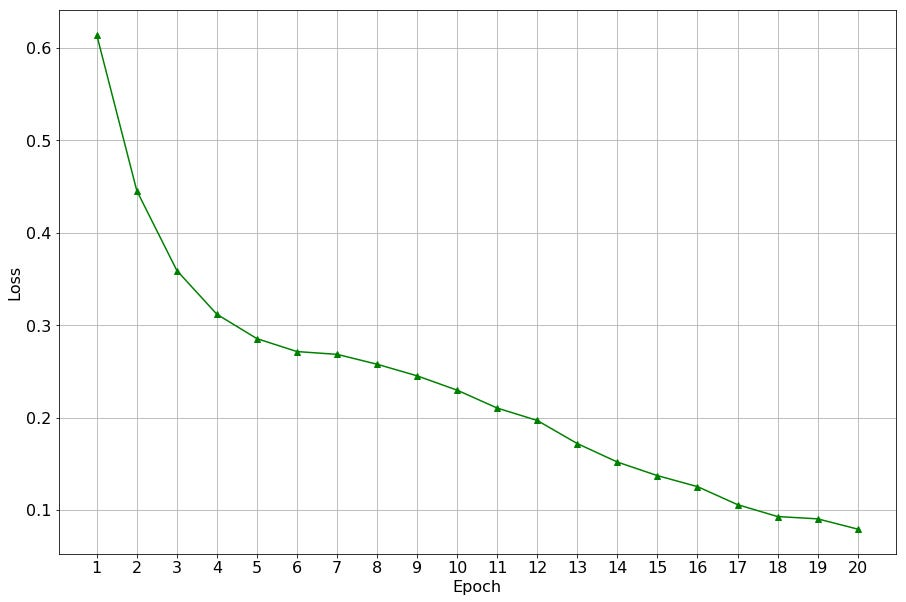Confusion Matrix and Training Loss for the Model with an Embedding Layer

Pre-trained Word Embeddings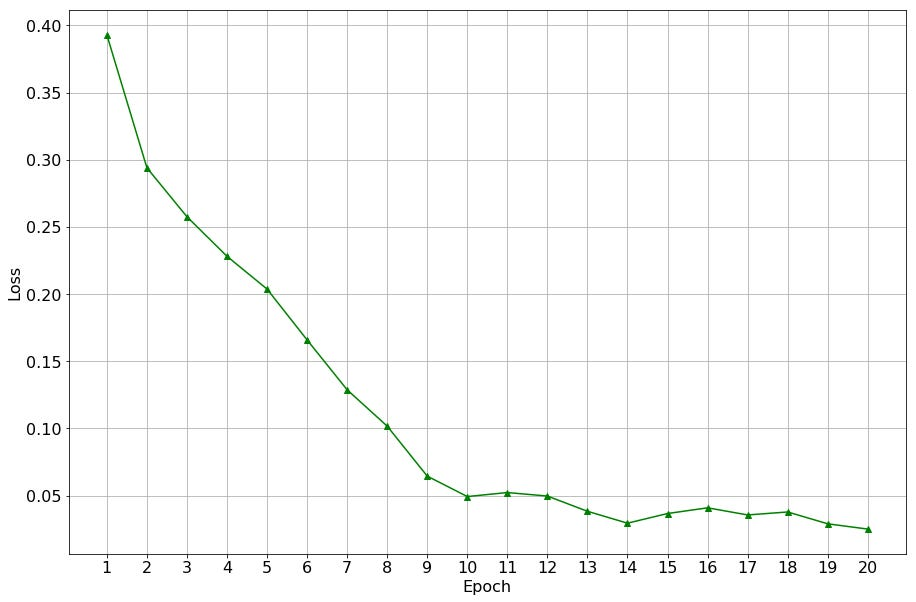Confusion Matrix and Training Loss for the Model with Pre-trained IMDB vectors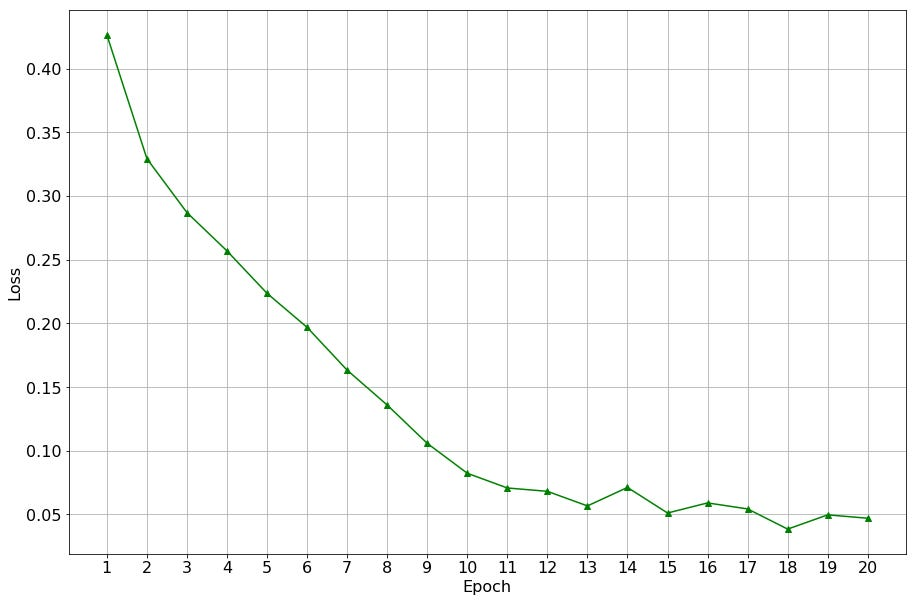Confusion Matrix and Training Loss for the Model with Pre-trained Glove vectors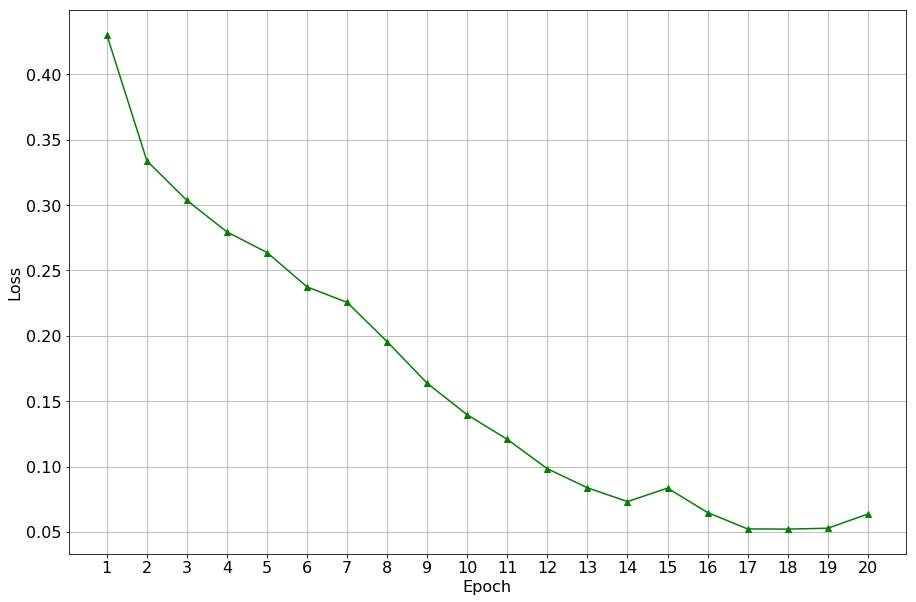Confusion Matrix and Training Loss for the Model with Pre-trained WIKI vectors

As seen above, the training loss decays more quickly for all three pre-trained embedding-based models compared to the embedding-layer-based model. Moreover, the precisions of the pre-trained embedding-based models are consistently higher for class 1. The overall precision and recall, as well as F1 score are presented in the following table. As seen, the pre-trained embedding-based models consistently outperform the embedding-layer-based model, albeit with a small margin.

``| Model | Precision (Macro) | Recall(Macro) | F1 ||------------------|-------------------|---------------|-------|| Embedding Layer | 85.6% | 85.8% | 85.6% ||------------------|-------------------|---------------|-------|| IMDB vectors | 86.1% | 86.1% | 86.1% || Glove 4B vectors | 86.4% | 86.4% | 86.4% || FT WIKI vectors | `86.0%` | `86.0`% | `86.0`% |``

Question Classification is the task of assigning a semantic class label to a question such that finding the answer to that question becomes easier. I used the TREC dataset from torchtext.datasets that comprises questions from 6 of the TREC classes, i.e. ABBREVIATION, ENTITY, DESCRIPTION, LOCATION and NUMERIC VALUE.

The following figures illustrate the training losses, as well as the performance of the test set in terms of confusion matrix, for different models:

Embedding Layer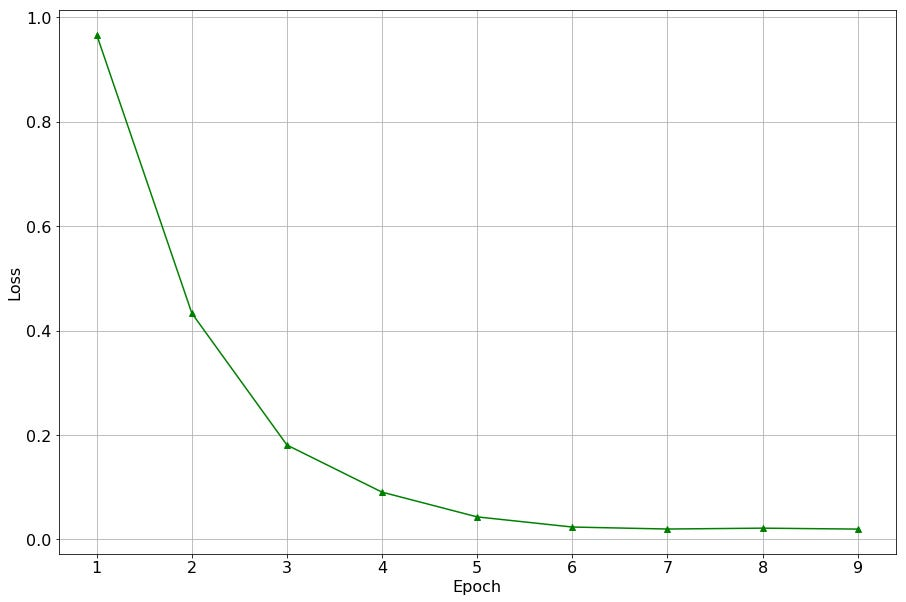Confusion Matrix and Training Loss for the Model with an Embedding Layer

Pre-trained Word Embeddings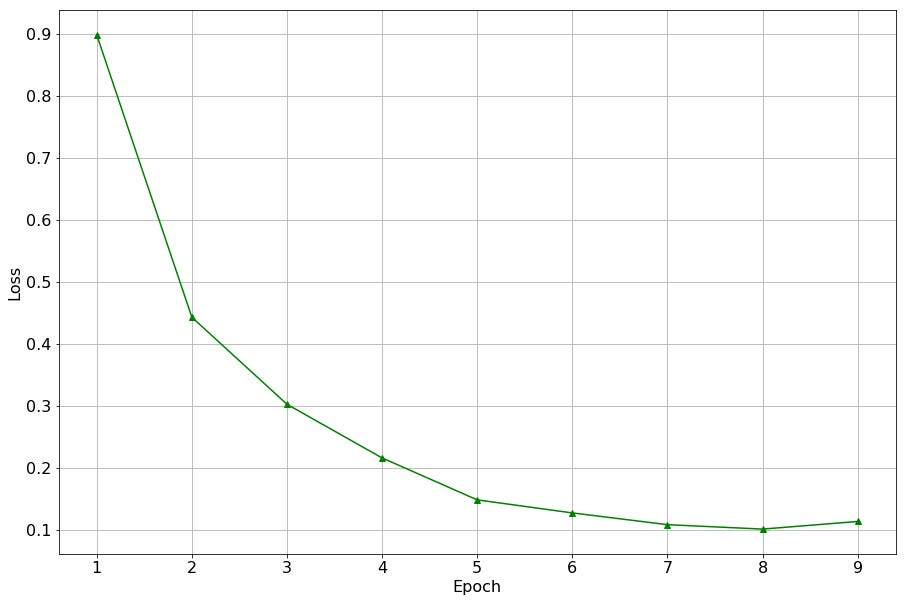Confusion Matrix and Training Loss for the Model with Pre-trained TREC vectors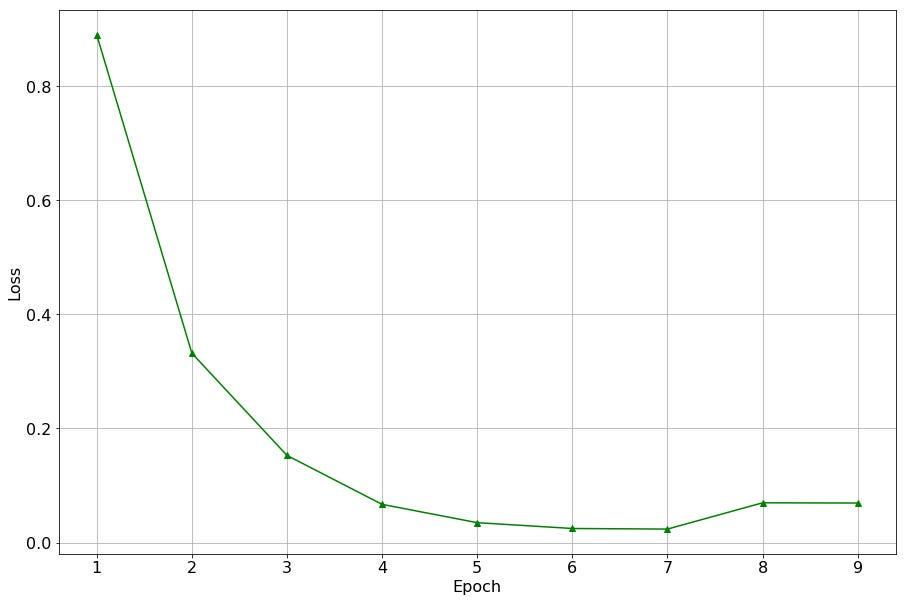Confusion Matrix and Training Loss for the Model with Pre-trained Glove vectors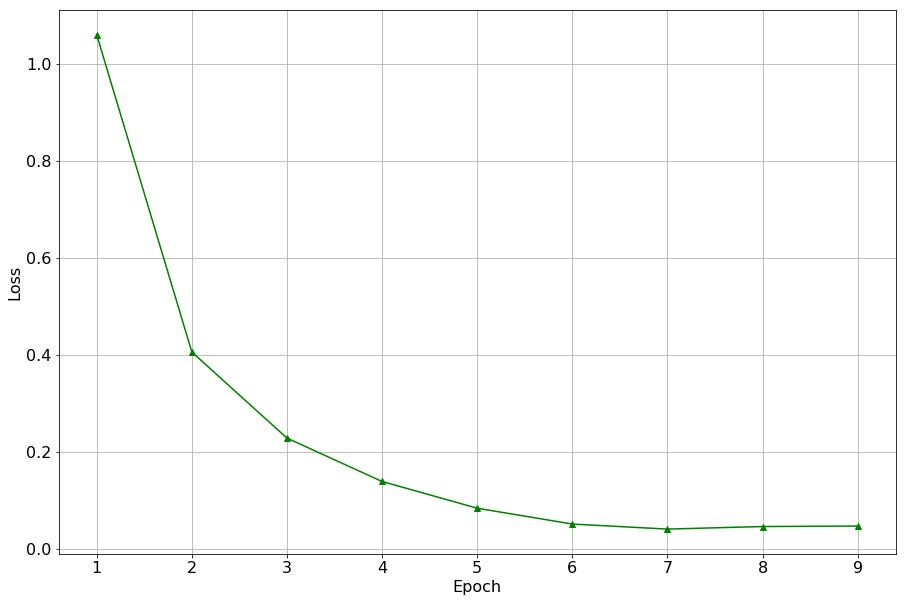Confusion Matrix and Training Loss for the Model with Pre-trained WIKI vectors

The training loss for the embedding-layer-based model and pre-trained embedding-based models decay relatively fast and without many fluctuations. No considerable differences between the training loss can be observed. The overall precision, recall and F1 score, on the other hand, improve for all pre-trained-embedding-based models except for the embeddings that were trained on the TREC question dataset. This is expected, since TREC is a small dataset with short questions and, hence, the vectors trained on this dataset will presumably not carry much semantic information. For the other pre-trained embedding-based models, i.e. Glove 4B and fastText WIKI, the performance considerably improves for several classes. See ABBR, for instance, where the percentage of correctly classified instances increases from 82% to 92-93%. Or LOC where the percentage of correctly classified instances increases from 84% to 90-96%. The overall precision, recall, and F1 score of the different models are presented in the following table.

``| Model | Precision (Macro) | Recall(Macro) | F1 ||------------------|-------------------|---------------|-------|| Embedding Layer | `87.2%` | `83.2%` | `84.8%` ||------------------|-------------------|---------------|-------|| TREC vectors | `86.1%` | `82.4%` | `83.8%` || Glove 4B vectors | `88.0%` | `86.7%` | `86.7%` || FT WIKI vectors | `87.0%` | `87.3%` | `87.0%` |``

### Take Home Lessons

Looking at the results of IMDB Sentiment Analysis task, it seems that pre-trained word embeddings lead to a faster training and a lower final training loss. It can be interpreted that the model could pick up more semantic signals from the pre-trained embeddings than it did from the training data through the embedding layer.

We see from the results of the TREC Question Classification task that vectors trained on a small corpus will have a worse performance than an embedding layer. However, vectors trained on a large corpus beat the embedding layer by a considerable margin in terms of both precision and recall.

Consistently for both tasks, precision and recall improve when we use pre-trained word embeddings (trained on a sufficiently large corpus). However, for the Sentiment Analysis task, this improvement was small, whereas for the Sentence Classification task, this improvement was much larger.

This can mean that for solving certain NLP tasks, when the training set at hand is sufficiently large (as was the case in the Sentiment Analysis experiments), it is better to use pre-trained word embeddings. But if they are not available, you can still use an embedding layer and expect comparable results. If, however, the training set is small, the above experiments strongly encourage the use of pre-trained word embeddings.

### Final Notes

Unlike imagery data that is directly acquirable in terms of real-valued numbers and, hence, is directly interpretable by machine learning models, textual data needs to be transformed into a representation, such that at least part of the extensive knowledge that each word bears can be carried into this representation. It is only then, that we can expect to have intelligent models that, to some extent, understand human language. Learning semantic representation for words has a relatively long history and it has been a core research topic in Linguistics and NLP. Word embeddings provide an efficient way of representing the words, however, their current capabilities are limited in terms of capturing the semantic, syntactic and collocational information that each word bears. That means there is still a lot of room for improvement when it comes to representing textual data, before we are able to develop intelligent systems that are able to understand and generate natural language at the text level.

For more of such posts, check out the omni:us medium publication!